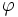# Golden Ratio

(Redirected from Golden ratio)

The golden ratio is a number, commonly known as phi (), defined to be the larger of the two solutions to. The value of phi isPhi is an irrational number. Its decimal expansion begins

1.61803...

The golden ratio was first defined by Euclid of Alexandria around 300BC. His definition reads:

A straight line is said to have been cut in extreme and mean ratio when, as the whole line is to the greater segment, so is the greater to the lesser.

## The golden ratio in nature and art

The golden ratio appears in innumerable places in nature and art. The periods of the planets are all related by the golden ratio. The proportions of the bodies of many animals, including humans, dolphins, and ants are all based on the golden ratio. The Parthenon, Egyptian Pyramids, The Last Supper, Ark of the Covenant, Noah's ark, and even the common credit card are all based on the golden ratio.

A dodecahedron, a solid in which each side is a pentagon, is related to the Golden Ratio in that both the surface area and the volume of a dodecahedron of unit edge length are functions of the Golden Ratio. Plato described the dodecahedron as the solid which the gods used for embroidering the constellation on the whole heaven.

## Fibonacci Numbers and the Golden Ratio

Leonardo of Pisa (c1170 – 1250), commonly known as Fibonacci, was an Italian mathematician, who wrote an arithmetic treatise called Liber Abaci ( the book of the abacus) in 1202. In this book, Fibonacci describes a problem concerning rabbit populations, the solution to which is the sequence:

1, 1, 2, 3, 5, 8, 13, 21, 34, 55, ...


The sequence can be continued by adding together the last two terms generated.

The numbers in the sequence are normally called Fibonacci numbers, and they have some remarkable properties, such as if the terms in the series are labelled with the natural numbers, then any Fibonacci number which occurs with a label which is prime, is itself prime. (for example, the 11th number in the sequence is 89. Both 11 and 89 are prime).

The relevance of this sequence here is that the ratio of the last two numbers generated at each stage in the Fibonacci sequence converges to the Golden Ratio. This can be checked:

• 1/1 = 1
• 2/2 = 2
• 3/2 = 1.5
• 5/3 = 1.6666..
• 8/5 = 1.6
• 13/8 = 1.625 etc.

It can be seen from this that successive ratios underestimate and overestimate the ratio, and the convergence is quite rapid.

For more on the sequence, see: http://www.mcs.surrey.ac.uk/Personal/R.Knott/Fibonacci/fibnat.html

## The golden ratio in art

It has been observed that the golden ratio is evident in the arrangement of branches along the stems of plants, and of veins in leaves. The golden ratio also has a place in art when used to determine what arrangements of a composition are aesthetically pleasing to the eye. Some would suggest that the "perfect" human face adheres to the golden ratio.

Leonardo da Vinci was a close friend of Luca Pacioli, who published a treatise (Divina Proportione) on the Golden Ratio in 1509. It has been claimed that he used the Golden Ratio in his composition of the Mona Lisa, but there is no documentation to prove this.

Salvador Dali deliberately used the golden ratio in his painting Sacrament of the Last Supper, incorporating also a huge dodecahedron around the supper table.

Le Corbusier designed an entire design system called the Modulor, based on the Golden Ratio, which aimed to give harmonious proportions to everything about a building.